## Wednesday, April 18, 2018

### A comment on the log-likelihood trace from Bayesian MCMC

I recently, as I have in the past, received an email from a concerned phytools user about the likelihood trace from one of phytools' handful of Bayesian MCMC methods: the function `ancThresh`.

They reported that instead of starting with a low likelihood value then climbing & stabilizing, the trace instead started with a relatively high value & then declined & stabilized. Uh-oh! Is this bad?

The answer is 'not necessarily.' In fact, `ancThresh` in particular tries to initiate the MCMC with values that make sense, given the data. I did this to try to make the MCMC more efficient, although this has been criticized by some purists (for reasons that I don't fully understand but that are most likely correct). When this is the case MCMC can sample perfectly efficiently from the posterior distribution but the samples have likelihood values that are (peculiar as this might seem) much lower than the likelihood of the very first sample of the chain!

In explaining this to the user I asked him to imagine a scenario in which we wanted to use Bayesian MCMC to estimate the mean of a normally distributed random variable from a sample. The ML value of the mean in this case is just the arithmetic mean, so if we started our MCMC with this value the likelihood trace will always go down before stabilizing. This becomes more & more exaggerated for more variable dimensions. If we have many variables in our model that we want our MCMC to simultaneously sample we may spend most of our time not particularly close to the ML, even though the mean from our posterior sample (assuming a relatively uninformative prior & a well-designed sampler) will be close to or the same as the ML estimate!

Rather than just say this, I thought it would be fun to show it. In the following I will conduct exactly the experiment that I described - first for one variable & then for various at the same time. To keep things simple I will concern myself only with the mean (or means) - ignoring the variances & covariances, which I assume we (somehow) know are all 1.0s and 0.0s, respectively.

First, our likelihood functions for a vector `x` or matrix `X`:

``````lik<-function(mu,x) sum(dnorm(x,mu,log=TRUE))
LIK<-function(mu,X) sum(mapply(lik,mu,split(t(X),1:ncol(X))))
``````

our prior functions:

``````prior<-function(mu,pm,pv) dnorm(mu,pm,sqrt(pv),log=TRUE)
PRIOR<-function(mu,pm,pv) sum(mapply(prior,mu,pm,pv))
``````

Now our MCMC sampler for one variable, vector `x`:

``````mcmc<-function(mu0,x,pm=0,pv=1e12,ngen,sample=100){
proposal<-0.1
l<-lik(x,mu0)
p0<-sum(prior(mu0,pm,pv)+l)
mu<-mu0
for(i in 2:(ngen+1)){
mu1<-mu[i-1]+rnorm(1,0,proposal)
l1<-lik(x,mu1)
p1<-sum(prior(mu1,pm,pv)+l1)
if((p1-p0)>log(runif(1))){
mu[i]<-mu1
l[i]<-l1
p0<-p1
} else {
mu[i]<-mu[i-1]
l[i]<-l[i-1]
}
}
ii<-seq(1,ngen+1,sample)
list(mu=mu[ii],l=l[ii],gen=ii)
}
``````

Let's imagine we have just one variable:

``````x<-rnorm(10)
x
``````
``````##   -2.3877360  0.6027703  0.9927019 -0.9601135  0.7277220  1.8369169
##   -0.8401388  1.5933389  0.0886217  1.3928630
``````

Now let's start our MCMC far from the ML estimate of the mean for `x`, which, as we know, is just the arithmetic mean:

``````obj<-mcmc(mu0=2,x,ngen=100000)
plot(obj\$gen,obj\$l,type="l",xlab="generation of MCMC",
ylab="log-likelihood",col="darkgrey")
``````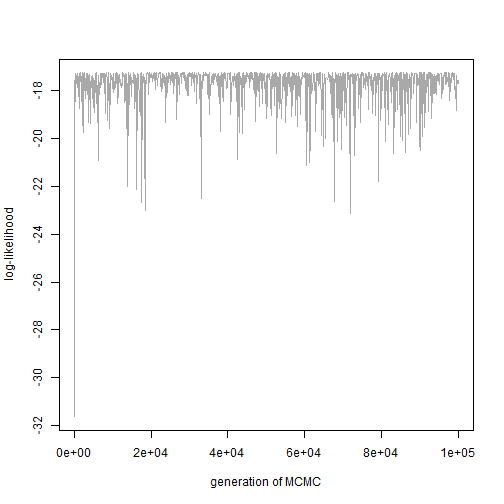This is probably what we were hoping to see & if we decided we'd converged to the posterior distribution perhaps we would exclude the first 20% of generations and estimate μ as follows:

``````ii<-ceiling(0.2*length(obj\$l))+1
mean(obj\$mu[ii:length(obj\$l)])
``````
``````##  0.3012088
``````

Which we would find is quite close to the ML value of μ:

``````optimize(lik,c(-1,1),x=x,maximum=TRUE)
``````
``````## \$maximum
##  0.3046946
##
## \$objective
##  -17.2593
``````

that, non-coincidentally, is precisely the same as the arithmetic mean of `x`:

``````mean(x)
``````
``````##  0.3046946
``````

But now what happens when we start with a value of μ equivalent (or, let's say, close to) it's MLE? Let's see:

``````obj<-mcmc(mu0=mean(x),x,ngen=100000)
plot(obj\$gen,obj\$l,type="l",xlab="generation of MCMC",
ylab="log-likelihood",col="darkgrey")
``````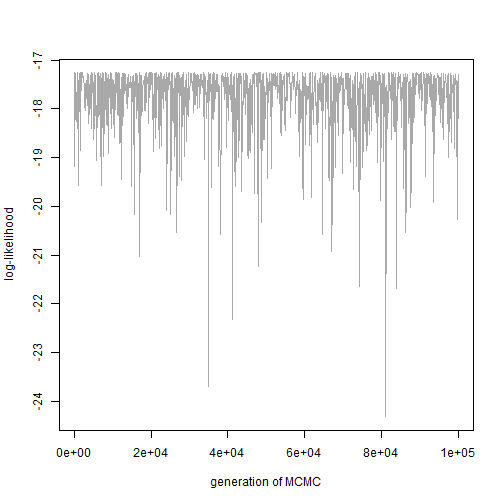Now, perhaps it's not obvious yet (because the chain spends most of its time pretty close to the MLE), but all values of the log-likelihood in the trace are less than or equal to the starting value. How do I know this? Because our starting value was the MLE.

Here, once again, we can see that we end up with a good estimate of μ regardless:

``````ii<-ceiling(0.2*length(obj\$l))+1
mean(obj\$mu[ii:length(obj\$l)])
``````
``````##  0.3061774
``````
``````mean(x)
``````
``````##  0.3046946
``````

Now let's make it a whole lot worse by increasing the parameterization of the problem. Here let's thus imagine an MCMC with 100 different variables to sample instead of 1.

``````MCMC<-function(mu0,X,pm=0,pv=1e12,ngen,sample=100){
proposal<-0.1
pm<-rep(pm,ncol(X))
pv<-rep(pv,ncol(X))
l<-LIK(mu0,X)
p0<-sum(PRIOR(mu0,pm,pv)+l)
MU<-matrix(NA,ngen+1,ncol(X))
MU[1,]<-mu0
for(i in 2:(ngen+1)){
mu1<-MU[i-1,]+rnorm(ncol(X),0,proposal)
l1<-LIK(mu1,X)
p1<-sum(PRIOR(mu1,pm,pv)+l1)
if((p1-p0)>log(runif(1))){
MU[i,]<-mu1
l[i]<-l1
p0<-p1
} else {
MU[i,]<-MU[i-1,]
l[i]<-l[i-1]
}
}
ii<-seq(1,ngen+1,sample)
list(mu=MU[ii,],l=l[ii],gen=ii)
}
X<-sapply(1:100,function(x) rnorm(10))
``````

If we start far from the MLE we see a similar pattern to before:

``````obj<-MCMC(mu0=rep(0.5,ncol(X)),X,ngen=100000)
plot(obj\$gen,obj\$l,type="l",xlab="generation of MCMC",
ylab="log-likelihood",col="darkgrey")
``````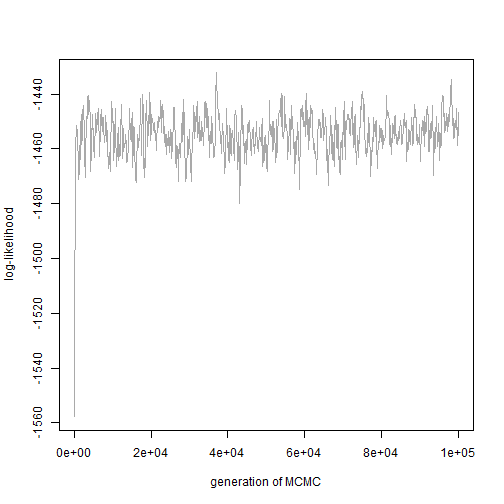and our estimates from our posterior sample should be highly similar to the MLEs:

``````ii<-ceiling(0.2*length(obj\$l))+1
plot(colMeans(X),colMeans(obj\$mu[ii:nrow(obj\$mu),]),pch=21,cex=1.5,
bg="grey",xlab="arithmetic means (MLEs)",
ylab="mean from post burn-in posterior sample")
abline(0,1,lwd=4,col=phytools::make.transparent("darkgrey",0.5))
abline(h=0,lty="dotted")
abline(v=0,lty="dotted")
``````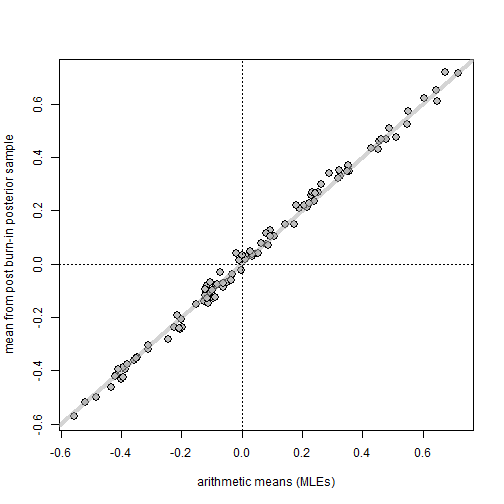On the other hand, if, as before, we start at the MLE we will see that our likelihood trace looks a lot different!

``````obj<-MCMC(mu0=colMeans(X),X,ngen=100000)
plot(obj\$gen,obj\$l,type="l",xlab="generation of MCMC",
ylab="log-likelihood",col="darkgrey")
``````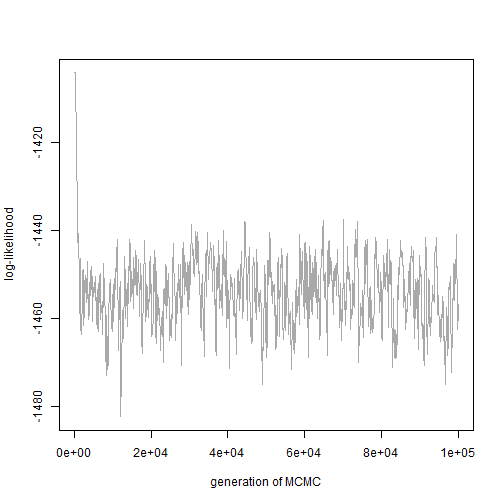Nonetheless, our estimates should be just as good as before, or better:

``````ii<-ceiling(0.2*length(obj\$l))+1
plot(colMeans(X),colMeans(obj\$mu[ii:nrow(obj\$mu),]),pch=21,cex=1.5,
bg="grey",xlab="arithmetic means (MLEs)",
ylab="mean from post burn-in posterior sample")
abline(0,1,lwd=4,col=phytools::make.transparent("darkgrey",0.5))
abline(h=0,lty="dotted")
abline(v=0,lty="dotted")
``````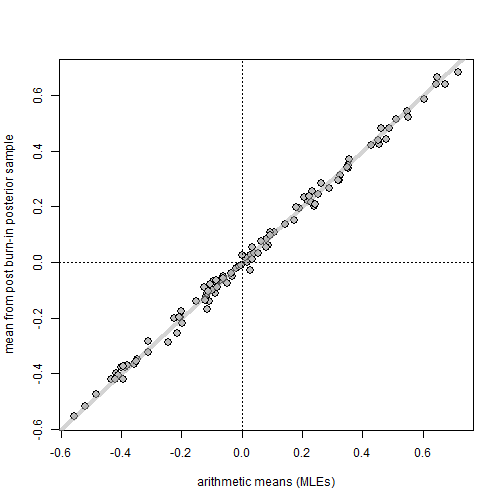This shows that for very high dimensional problems the MCMC can spend most, if not all, of its time in regions of parameter space that have quite low likelihood, but still whilst all the while sampling from the posterior distribution such that our estimates at the end are just fine.